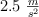# Relationship between linear and angular quantities (7425)

, por

A car that starts fromand uniformly accelerates atfor 5 s.

a) Calculate the final velocity and the displacement of the car.

b) What is the diameter of the tire if the angular displacement is 60 rad?

c) What is the final angular velocity and angular acceleration of the tires?

## SOLUCIÓN:

a) The equation for calculating the final velocity is:Just replace the data and calculate:The equation for calculating the displacement is:Using the data in the problem statement:b) You must relate the distance covered to the radius of the wheel:Diameter is two times the radius:c) The final angular velocity is:The angular acceleration is:Descarga el enunciado y la resolución del problema en formato EDICO si lo necesitas.Buscar en esta web

Por palabras clave

Por temas

Búsqueda libre